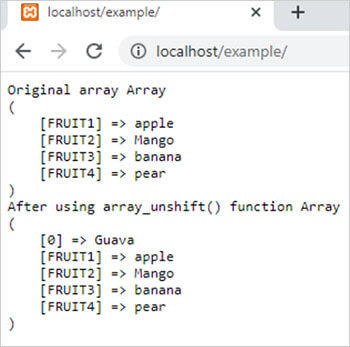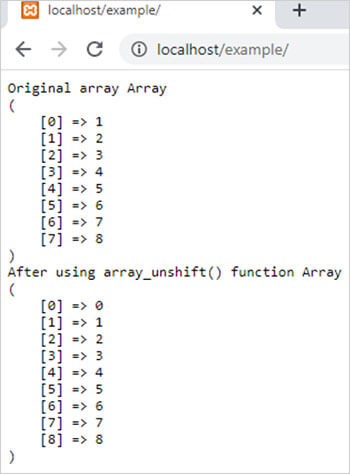array_unshift() function in php || PHP Array Unshift Functions

# array_unshift() function in php

0 1485

array_unshift() function of PHP used to shift the elements of array one position in right means it inserts one or more new elements into the starting of a PHP array.

If the array is an index array then it re-indexed the array from 0. It is an inbuilt function of PHP.

Syntax:

array_unshift(\$array,\$value1,\$value2,.....,\$valueN);

here,

\$array is a PHP array and \$value1,\$value2 and \$valueN are values to be added to the \$array.

Example 1:

```<?php
\$arr1=array("FRUIT1"=>"apple","FRUIT2"=>"Mango","FRUIT3"=>"banana","FRUIT4"=>"pear");
echo "<pre>";
echo "Original array ";
print_r(\$arr1);
array_unshift(\$arr1,"Guava");
echo "After using array_unshift() function";
print_r(\$arr1);
?>```

Output:Example 2:

```<?php
\$arr1=array(1,2,3,4,5,6,7,8);
echo "<pre>";
echo "Original array ";
print_r(\$arr1);
array_unshift(\$arr1,"0");
echo "After using array_unshift() function ";
print_r(\$arr1);
?>```

Output:Share: